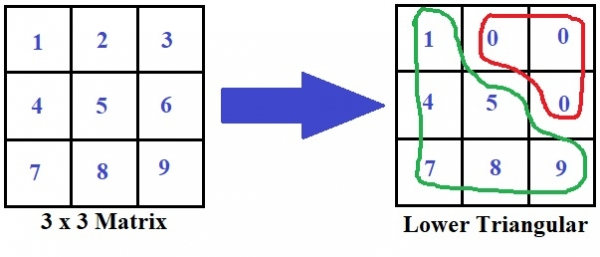# Print lower triangular matrix pattern from given array in C Program.

Given with the matrix of n x n the task is to print that matrix of n x n in lower triangular pattern.

Lower triangular matrix is a matrix which has elements below the principle diagonal including the principle diagonal elements and rest elements as zero.

Let’s understand this with help of a diagram −Above the elements in green are the elements below the principle diagonal and the red elements are the elements above the principle diagonal which are set as zero.

## Example

Input: matrix = {
{ 1, 2, 3 },
{ 4, 5, 6 },
{ 7, 8, 9 } }
Output:
1 0 0
4 5 0
7 8 9

## Algorithm

int lower_mat(int mat[n][m])
START
STEP 1: DECLARE I AND j
STEP 2 : LOOP FOR i = 0 AND i < n AND i++
LOOP FOR j = 0 AND j < m AND j++
IF i < j THEN,
PRINT "0\t"
ELSE
PRINT mat[i][j]
END IF
END FOR
PRINT newline
END FOR
STOP

## Example

#include <stdio.h>
#define n 3
#define m 3
int lower_mat(int mat[n][m]){
int i, j;
for ( i = 0; i < n; i++){
for ( j = 0; j < m; j++){
if( i < j )
printf("0\t");
else
printf("%d\t", mat[i][j]);
}
printf("");
}
}
int main(int argc, char const *argv[]){
int mat[n][m] = {
{1, 2, 3},
{4, 5, 6},
{7, 8, 9}
};
lower_mat(mat);
return 0;
}

## Output

If we run above program then it will generate following output −

1 0 0
4 5 0
7 8 9